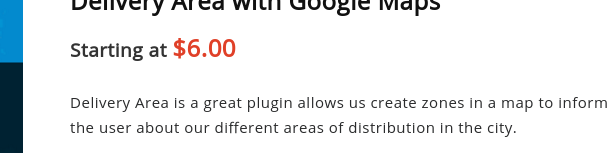# Customize the view of the price range in Woocommerce 3.2.x

Many times when customizing a variable product we have found that Woocommerce paints the price range from the lowest value to the highest value in a not so pretty way.

In this example we will customize the price in this way: “From \$12” or “Starting at \$45” or the thousands of alternatives that we can think of.

There are many hooks that we can use for these cases, because if we see the function get_price_html() we notice that we have to choose, however we will take the most common of all, the hook woocommerce_get_price_html .

## Let’s start

```<?php

function lets_get_price_html(\$price, \$product ) {

if( !\$product->is_type( 'variable' ) )
return \$price;

\$prices = \$product->get_variation_prices( true );

\$min_price = current( \$prices['price'] );
\$max_price = end( \$prices['price'] );

// Return price if min is equal to max.
if ( \$min_price === \$max_price ) {
return \$price;
}
return sprintf( __( 'Starting at %s', 'gowoo' ), wc_price( \$min_price ) . \$product->get_price_suffix() );
}
?>```

## Explanation

The product has 2 parameters, the first one is the classic price range with all HTML and the second one is the \$product global object, remember that it must be called in this way, otherwise you will get an error.

We make a conditional if (! \$ Product-> is_type (‘variable’))  since this function only applies to variable products

We obtain the active variations and bring the minimum and maximum price found at the beginning and end of the \$prices array respectively.

If in case the minimum and maximum are equal then it is not a variable product, so we return the same value that was sent to us.

And finally we return what we want. I want to emphasize that the wc_price() function wraps it in HTML tags to make it look cool.

### Result